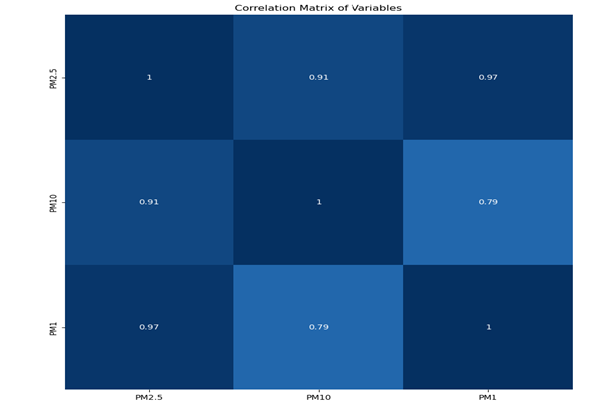top of page
Search

# City of Chicago Air Quality Exploration & Design of an AI Neural Network Model

Updated: Oct 14

Mentor: Kiran Palla

Anirudh Chinthagunta, AI Intern, AVS Academy, IL

Project Summary

While going through the DeepLearning.AI course for AI for Good in Public Health, the team analyzed the air quality data for the city of Bogota, Columbia. The goal of that learning exercise was to find the missing pollutant values from city censors using AI neural network method.

A use case was implemented for the city of Chicago's air pollution data. The goal of the project is to estimate missing pollutant values using an AI neural network model.Data is downloaded from purpleair.com for two sensors in the city of Chicago for June, July, August 2023.

Sample Data

A sample of rows are shown below from the dataset:Plot histograms of different pollutants

A Histogram is plotted to show the distribution of pollutant measurements. As seen in the chart, pollutants have an uneven distribution skewing towards lower values less than 30 μg/m3 which is desirable and they have very few values more than 100μg/m3.

This indicates that the air pollution in the city of Chicago is in the desirable range most of the time at this sensor.Construct a correlation matrix to quantitatively look for correlation.

Different pollutants like PM2.5, PM10, PM1 have a positive correlation with each other meaning they increase or decrease in the same direction. A value of PM10 can be used to fill in a missing value of PM2.5. This is possible when we can calculate a correlation value and the below correlation matrix shows correlation values between various pollutants in a grid pattern. PM10 and PM1 have high positive correlation values of 0.91 and 0.91, indicating their values can be used to fill in missing PM2.5 values.Visualize Pollutant measurements over time.

A time series plot is shown with PM2.5 values over the time period available and we can see that there are gaps in the data, which are missing PM2.5 values.Time series plot below shows the distribution of PM2.5 values over the hour of day and the hourly average is well above the recommended level. The shaded region at any given hour represents the minimum and maximum values for that hour of the day.Patterns observed from data analysis:

● Pollutant levels change depending on time of day or day of week.

● Pollutant levels change relative to each other.

● Pollutant correlations can be measured quantitatively by correlation values.

● An AI neural network model can learn from these patterns in data and make better predictions of missing pollutant values.

Visualize the distribution of gaps in the data

The next plot shows counts of gap size in hours (horizontal axis) vs. number of missing data points due to gaps of that size (vertical axis). This plot gives a sense of how different sized gaps in the data are contributing to the missing data problem. For example, there are 24 gaps of 2 hours and 16 gaps of 7 hours in the data.

Total missing values for each pollutant:Train and test a neural network model for estimating missing PM2.5 data

• A neural network, at its core, is a model with neurons in layers which takes in inputs and predicts outputs.

• Outputs from the first layer of neurons become inputs for the next layer of neurons.

• An artificial neural network can learn correlations in the data which might be a little hard to capture with rule based systems or a simple linear relationship.

• The neural network is trained to learn how PM2.5 values are related to values for all the other pollutants as well as time of day, day of week, and sensor location.

• Training a neural network is an iterative process and with each iteration the neural network becomes better at making predictions.

• The trained neural network is run with the input data file and the missing PM2.5 values are estimated.

A sample of the data is shown below and the flag columns include different values:

None where there is original data, 'neural network' where the PM2.5 values were imputed with the neural network.Visualize the results of filling in missing PM2.5 values

The same time series plot is shown below with the imputed PM2.5 gaps shown in red and closely match the real values.133 views
bottom of page